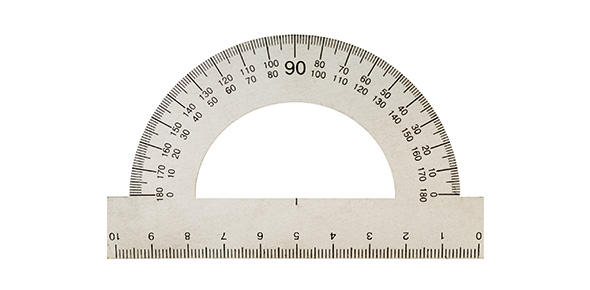# Unit 4 Conversions Quiz

29 Questions | Attempts: 3203
ShareSettingsMemorizing Conversions

• 1.
• 2.
1 minute equals ________ seconds
• 3.
1 hour equals ________ minutes
• 4.
1 day has _______ hours
• 5.
1 week has ______ days
• 6.
1 month has approximately ______ weeks
• 7.
1 year usually has _______ days
• 8.
1 year has approximately _____ weeks
• 9.
1 year has ____ months.
• 10.
1 decade is equal to ______ years
• 11.
1 century is equal to _______ years
• 12.
100 years is equal to _______ decades
• 13.
1 meter (m) equals _______ centimeters (cm)
• 14.
1 meter (m) equals ______ millimeters (mm)
• 15.
1 kilometer (km) equals ________ meters (m)
• 16.
1 centimeter (cm) equals _______ millimeters (mm)
• 17.
1 foot (ft) equals _________ inches (in.)
• 18.
1 yard (yd) equals __________ inches (in.)
• 19.
1 yard (yd) equals _________ feet (ft)
• 20.
1 mile equals _________ feet (ft)
• 21.
1 cup (c) equals _________ fluid ounces (fl oz)
• 22.
1 pint (pt) equals ________ cups (c)
• 23.
1 quart (qt) equals _______ pints (pt)
• 24.
1 gallon (gal) equals ________ quarts (q)
• 25.
1 pound (lb) equals _________ ounces (oz)

## Related TopicsBack to top
×

Wait!
Here's an interesting quiz for you.